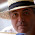## 10 August 2014

### Quiz 1: Find sum of all the numbers using ARGV.

Problem:
Give sum of all the numbers passed as command like argument.

Sample Input:
5 6

Sample Output:
Sum is 11

Solution:

my \$tmp = 0;
my \$sum = 0;
my \$len = @ARGV;
while(\$tmp < \$len)
{
\$sum = \$sum+\$ARGV[\$tmp];
++\$tmp;
}
print "Sum is \$sum";

Tips:

• @ARGV stores all command like arguments in array ie for input 5 6 , \$ARGV = 5 and \$ARGV = 6.

#### 3 comments:

1.2.my \$sum;
\$sum += \$_ for @ARGV;
print "Sum is \$sum\n";

3.@Dave: For 0, your solution will give blank sum, use \$sum=0;